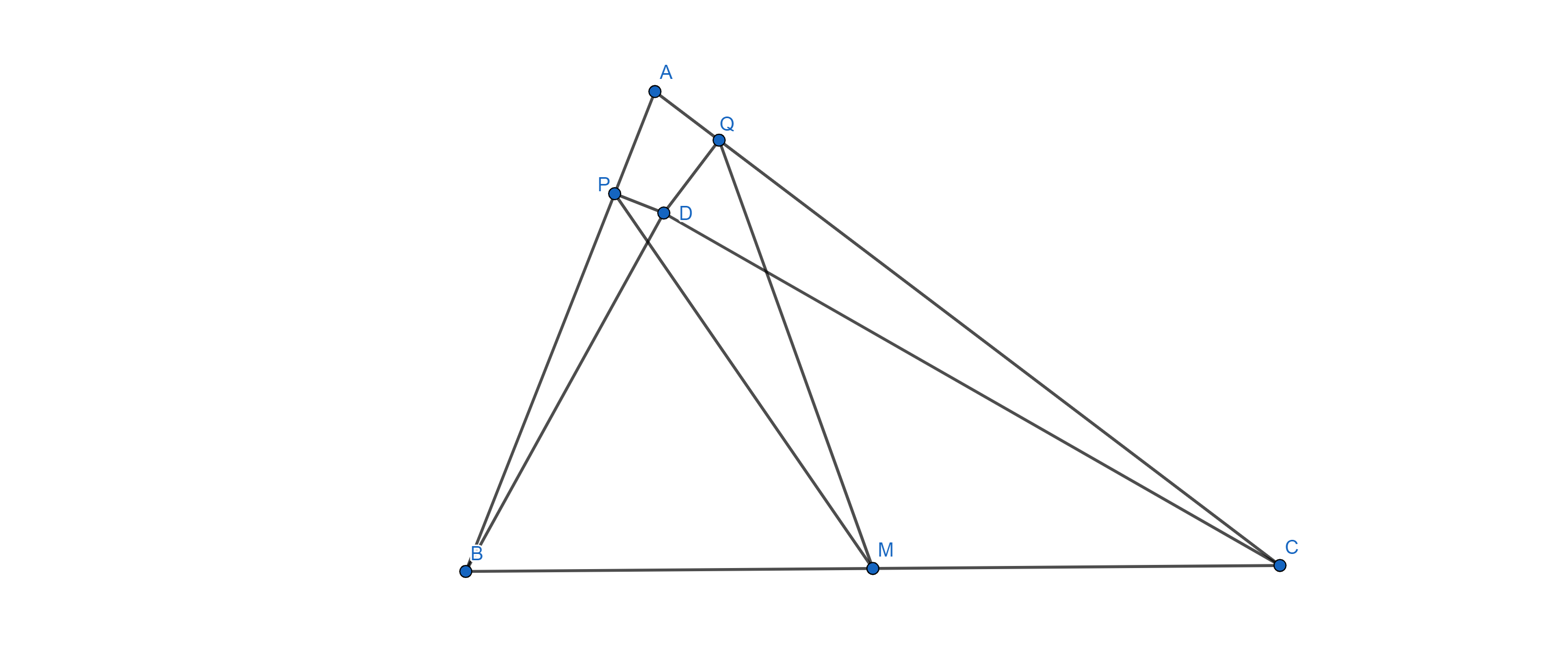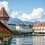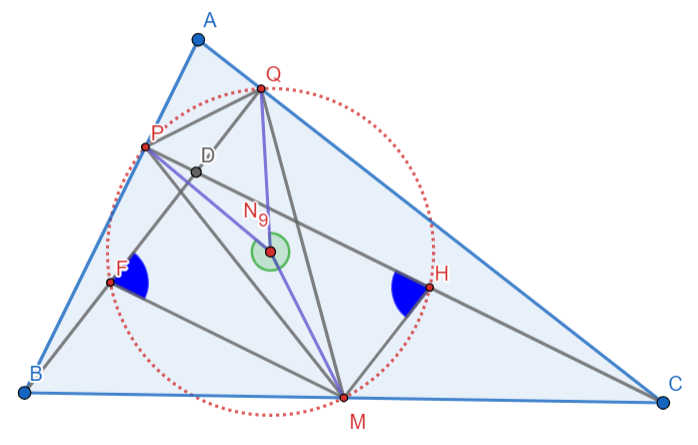# Prove itSuppose that P and Q are points on the sides AB and AC respectively of △ABC. The perpendiculars to the sides AB and AC at P and Q respectively meet at D, an interior point of △ABC. If M is the midpoint of BC, prove that PM = QM if and only if ∠BDP=∠CDQ .

I tried to come up with a solution using Euclidean geometry only, but to no avail.Note by Abu Nazam Sk Md Tamimuddin
7 months, 1 week ago

This discussion board is a place to discuss our Daily Challenges and the math and science related to those challenges. Explanations are more than just a solution — they should explain the steps and thinking strategies that you used to obtain the solution. Comments should further the discussion of math and science.

When posting on Brilliant:

• Use the emojis to react to an explanation, whether you're congratulating a job well done , or just really confused .
• Ask specific questions about the challenge or the steps in somebody's explanation. Well-posed questions can add a lot to the discussion, but posting "I don't understand!" doesn't help anyone.
• Try to contribute something new to the discussion, whether it is an extension, generalization or other idea related to the challenge.

MarkdownAppears as
*italics* or _italics_ italics
**bold** or __bold__ bold
- bulleted- list
• bulleted
• list
1. numbered2. list
1. numbered
2. list
Note: you must add a full line of space before and after lists for them to show up correctly
paragraph 1paragraph 2

paragraph 1

paragraph 2

[example link](https://brilliant.org)example link
> This is a quote
This is a quote
    # I indented these lines
# 4 spaces, and now they show
# up as a code block.

print "hello world"
# I indented these lines
# 4 spaces, and now they show
# up as a code block.

print "hello world"
MathAppears as
Remember to wrap math in $$ ... $$ or $ ... $ to ensure proper formatting.
2 \times 3 $2 \times 3$
2^{34} $2^{34}$
a_{i-1} $a_{i-1}$
\frac{2}{3} $\frac{2}{3}$
\sqrt{2} $\sqrt{2}$
\sum_{i=1}^3 $\sum_{i=1}^3$
\sin \theta $\sin \theta$
\boxed{123} $\boxed{123}$

Sort by:

$PM=QM$ is a direct result of the Nine-Point Circle Theorem

- 7 months ago

But P and Q are given to be random points on AB & AC respectively. And yes, the result holds when D is the orthocentre.- 7 months ago

But points P and Q are given to be random which indicates that points P, D and C should not be collinear necessarily. Same thing applies for points Q, D and B.

Yes, but when the angles are equal, doesn't it imply that they are collinear (vertically opposite angles) ?

- 7 months ago

No, not necessarily. It's still possible for them to be equal without being intersected in lines.

I found a solution here. https://math.stackexchange.com/questions/3961588/geometric-problem-concerning-relation-of-equal-segments-and-angles

- 6 months, 3 weeks ago

That was a nice problem!

- 6 months, 3 weeks ago

Indeed!

- 6 months, 3 weeks ago

test